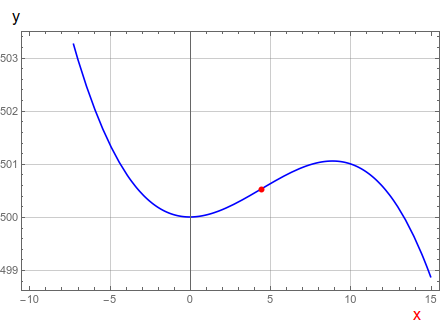# The total sales S, in thousands of dollars, of a certain company is related to the amount of...

## Question:

The total sales S, in thousands of dollars, of a certain company is related to the amount of money the company spends on advertising its products by the function

{eq}S = -0.003x^3 + 0.04x^2 + 500 {/eq} with {eq}0 \leq x \leq 200 {/eq}

where x is measured in thousands of dollars. Is this sales function (strictly) concave everywhere? Explain your answer carefully using both calculus and diagram.

## Concavity:

The concavity is a measure of the behavior of curves. It is related to the second derivative of the function and it is concave up (down) when the second derivative is positive (negative). Visually speaking, it can be related to the direction in which the curve develops. The concavity changes in the points of inflection, which met that the second derivative is zero.

To find the intervals of concavity we use the second derivative:

{eq}\begin{align} S(x) &= -0.003x^3 + 0.04x^2 + 500\\ S''(x) &= -0.018x + 0.08\\ \\ S''(x)&=0\Rightarrow\;\;\;x=4.44\\ \\ x&<4.44\Rightarrow\;\;\;S''(x)>0\\ x&>4.44\Rightarrow\;\;\;S''(x)<0\\ \end{align} {/eq}

Then the funcion is not strictly concave up everywhere, just in the interval:

{eq}-\infty<x<4.44 {/eq}

In Figure 1 a graph of the function is presented:The red point indicates the inflection point {eq}x=4.44 {/eq}, where the function changes from concave up to cancave down.